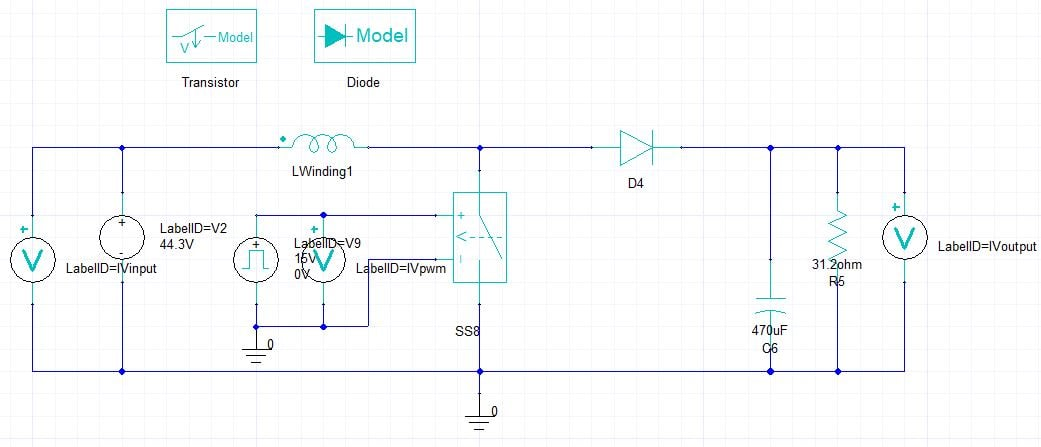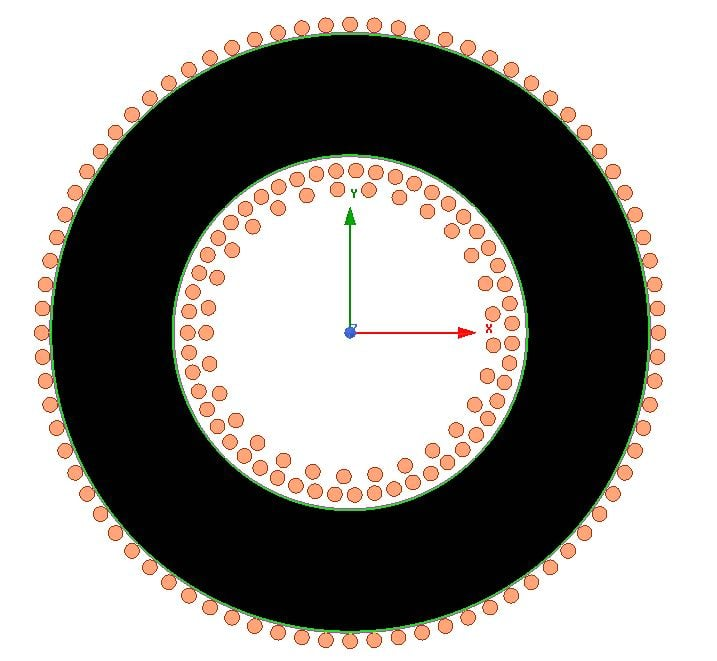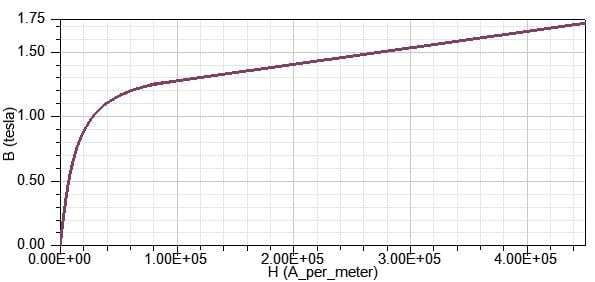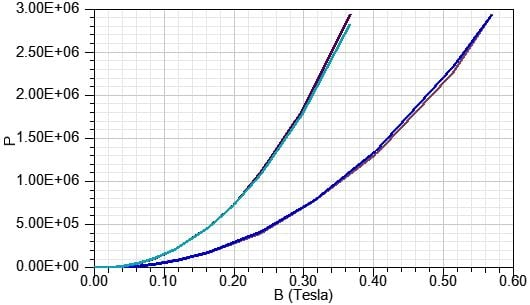# Power Ferrite Core Loss model use of Kdc (Maxwell Core Loss With DC Bias)

Member Posts: 1

Hello Ansys Community.

I intend to reproduce inductor core losses of experimental results using Maxwell transient simulation. I have obtained the core losses using the B-H loop method (a.k.a. Two-wire method). The experiment was performed using a boost converter, so variations of current ripple, DC Bias and frequency could be made.

To achieve this, I setup the following Maxwell project:

• A Maxwell Circuit simulation simulates the Boost Converter. The circuit is excited with the values of electrical measurements. The inductor of the circuit is assigned as an external winding (Maxwell 2D);
• In Maxwell 2D I set the excitation to be the external circuit.
• To characterize the material, I used experimentally measured B-H curve to set the relative permeability.
• Core loss data (BP Curve) was set using manufacturer provided curves for two frequencies, 10kHz and 20kHz, for which the best fit is the Power Ferrite core loss model. The Steinmetz parameters obtained by Maxwell matched the manufacturer provided ones well. My measurements are in 10kHz.

The project reproduces reality very well: the output voltage matches the measurements, and so does the RMS and ripple current in the inductor. The problem is in core losses with DC Bias.

As far as I understand, the core loss curves provided by the manufacturer are measured given a sinusoidal excitation, and so should be the curves we provide Maxwell with. I believe that maxwell works out the whole non-sinusoidal excitation part by itself.

The manual isn't very informative on DC-DC converter simulation, and the nature of the inductor waveforms (Nonsinusoidal + DC Bias) isn't easily covered by models. This is where the guessing starts.

1. I assume that the B in the BP curve we provide Maxwell with is actually the Peak value of B. Given the DC Bias of my scenario, I guessed that Maxwell understands it as: Bpk = ΔB/2. Am I correct? At least this is what matches manufacturer data. I could not find information about this on the manual.

2. In the user manual, on Section "Assigning Materials 9-39", the equation through which the term Kdc affects the Electrical Steel core loss model is presented, but I haven't been able to find anything about the Power Ferrite core loss model. In fact, I couldn't even find the equation for Power Ferrite core loss model, but I have guessed that it is: Pv = Cm * f^x * B^y.

So how does the Kdc term comes into play here? I have been trying to guess how it works by trial and error, mainly assuming that it works exactly like it does for the Electrical Steel core loss model, as a simple multiplier inside the Cdc term, which would then multiply the Pv equation. Can I have confirmation on this?

3. Also, I am aware that the program has an automated way of determining Kdc if I just leave it at zero, but then the results don't agree with experimental data. I have also tested and confirmed that if I put in a very small value for Kdc, say, 1E-10, the results agree with experimental data for low DC Bias. It does act different that just leaving it at zero, which led me to believe that the use of Kdc for the Power Ferrite core loss model would be similar to the Electrical Steel core loss model.

4. My approach to determine the weight of DC Bias on material core loss is fitting the experimental results, but then again, without confirmation of how Kdc affects the Pv equation for Power Ferrite, it is difficult to work it out.

The main question here then is:

How is the "Power Ferrite" core loss model affected by Kdc?

## Comments

• edited November 2019

Here are some pictures that wouldn't fit the main post.

• The Maxwell Circuit Model:• The Maxwell 2D Transient Model:• The BH Curve:• The BP Curves• Hello @pbolsi ,

How did you manage to find Kdc?

Regards.

Sign In or Register to comment.